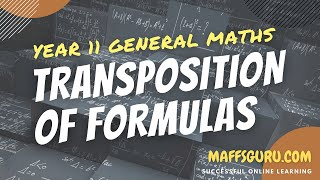Transposition of formulasSorry!

This video is for subscribers only.

To view this video please support me by purchasing a (very cheap!) years access.

Videos in this series
Please select a video from the same chapter
Chapters
Gain access to chapters by taking out a (very reasonable and cheap!) one year plan
Transposition of formulas
Linear relations and equations
Year 11
Powering through the videos for the Year 11 (VCE) General Maths Units 1 and 2 course looking at Linear Equations and Relations. It doesn't get any better than this! Having already looked at how we can use the CAS to solve equations and using "by hand" methods to solve, we find that the CAS can also transpose equations for us. It's exactly the same as the things we have already been doing. So, I take the time, in this video, to look at how to use the CAS to transpose formulas and then solve them. There are a number of worked examples all explained in my unique style. Seriously ... I've got your back. So, why not try me.
Published: April 6, 2021
Length: 07 mins and 40 seconds
Lesson notes

There are currently no lesson notes at this time to download. I add new content every week.
Exam resources

There are currently no associated exam questions for this topic.

Video tags

vce maths maths tutorials Transposition of formulas vce general maths general maths unit 1 and 2 maths linear equations linear relations transposing equations transposing math equations transposing formulae year 11 maths grade 11 maths how to transpose a formula using the CAS to transpose
LEGAL STUFF

Where videos relate to VCE and I have used VCAA questions the following should be noted:
VCE Maths exam question content used by permission, ©VCAA. The VCAA is not affiliated with, and does not endorse, this video resource. VCE® is a registered trademark of the VCAA. Past VCE exams and related content can be accessed at www.vcaa.vic.edu.au